### Towards Green Energy Class 10th Science And Technology Part 2 MHB Solution

##### Class 10th Science And Technology Part 2 MHB Solution

###### Exercise
Question 1.

Remake the table taking into account relation between entries in three columns.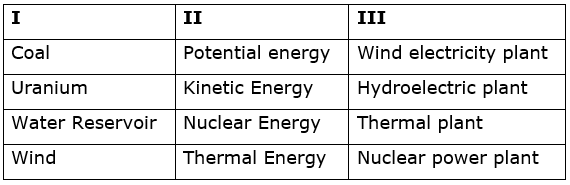Question 2.

Which fuel is used in thermal power plant? What are the problems associated with this type of power generation?

Coal is used as fuel in thermal power plant.

The problems associated with thermal power generation are:

1. Air pollution – due to burning of coal, harmful gases like carbon di oxide, Sulphur oxide and nitrogen oxide is released which pollute the environment.

2. Health problems – soot particles and the harmful gases like carbon dioxide, Sulphur oxide and nitrogen oxide released from burning coal may cause health issues related to respiratory system.

3. Limited resources – coal is a non-renewable source of energy which means that the amount of coal present in earth is limited. Thus, there will be limitations in the usage of coal if not used cautiously and effectively now.

Question 3.

Other than thermal power plant, which power plants use thermal energy for power generation? In what different ways is the thermal energy obtained?

1. Nuclear power plants: in nuclear power plants, the nuclear energy is obtained when uranium atoms are bombarded with neutrons. The uranium atoms break into smaller atoms through a process of fission, hence releasing nuclear energy. The nuclear energy obtained is converted into thermal energy. The kinetic energy of steam obtained through this is converted into kinetic energy of turbine which thus helps us obtain electrical energy.

2.Solar thermal power plant: Thermal energy is obtained from solar radiation in solar thermal power plants. Many reflectors reflect and concentrate solar radiation on absorbers. There, the solar energy is converted into thermal energy. The thermal energy obtained from solar radiation is used to drive the turbine which thus helps in the generation of electricity in solar thermal power plants.

Question 4.

Which type/types of power generation involve maximum number of steps of energy conversion? In which power generation is the number minimum?

NUCLEAR POWER PLANT involves maximum number of steps in the process of transformation.
1. First, nuclear energy is released by fission of nuclei of atoms like uranium or plutonium.
2. The nuclear energy released is used to generate steam (thermal energy) of high temperature and high pressure.
3. The thermal energy is transformed into kinetic energy in steam.
4. The kinetic energy in steam, is then converted into kinetic energy in the turbine.
5. The turbine rotates hence generating electrical energy.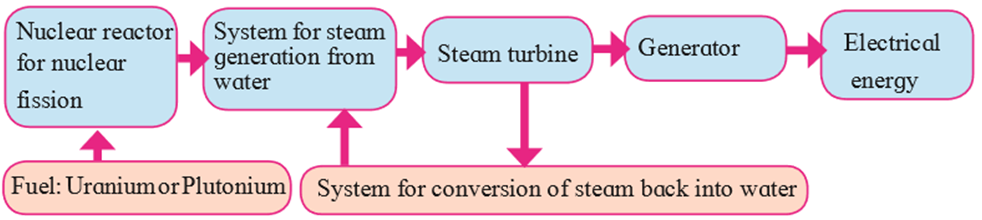ELECTRIC GENERATOR USING WIND involves the minimum steps in the process of transformation.
1. The kinetic energy in the wind is used in wind power plants
2. This kinetic energy is converted to kinetic energy in the wind-turbine. Wind-turbine is a machine which converts the kinetic energy of the wind to electrical energy.
3. The turbine rotates hence generating electrical energy.Question 5.

Solve the following crossword puzzle:

a. Maximum energy generation in India is done using ..... Energy.

b. ...... energy is a renewable source of energy

c. Solar energy can be called.... energy.

d. .... energy of wind is used in wind mills.

e. ..... Energy of water in dams is used for generation of electricity.

a. Maximum energy generation in India is done using thermal energy.

Explanation: Thermal energy can be obtained using various fuels like water, nuclear compounds, solar energy. Thus thermal energy contributes to the maximum energy generation in india.

b. Wind energy is a renewable source of energy.

Explanation: There is a limitless supply of wind and wind energy is collected from sources which are replenished naturally. Thus, making wind energy a renewable source of energy.

c. Solar energy can be called green energy.

Explanation: Solar energy is obtained from sunlight, which is a renewable source of energy. Also, electricity obtained through solar energy does not cause any negative impact on the environment. Solar energy is an environmental friendly form of energy and is thus called green energy.

d. Kinetic energy of wind is used in wind mills.

Explanation: The kinetic energy in the wind is used in wind power plants.
This kinetic energy is converted to kinetic energy in the wind-turbine, which rotates and produces electricity.

e. Potential energy of water in dams is used for generation of electricity.

Explanation: The potential energy in water stored in dam is converted into kinetic energy of water which drives the turbine, hence generating electrical energy.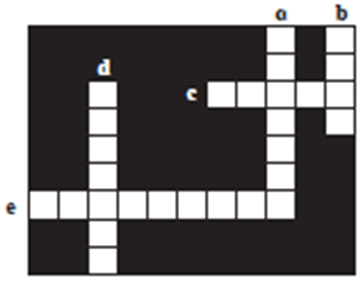Question 6.

Explain the difference:

Conventional and Non-conventional Sources of energy.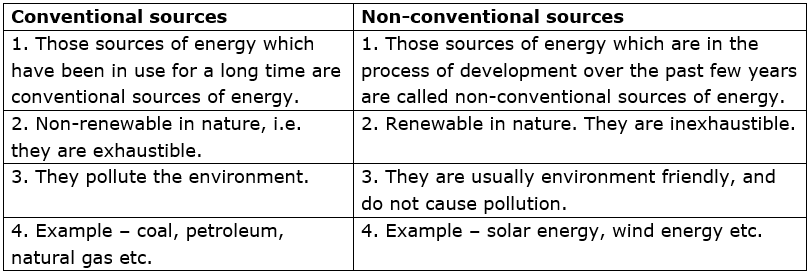Question 7.

Explain the difference:

Thermal electricity generation and solar thermal electricity generation.

Thermal energy –

1. Coal and nuclear fuel is used to generate electricity.

2. The thermal energy released due to burning of fuel produces steam at very high temperature and pressure. This energy in the steam drives the turbine.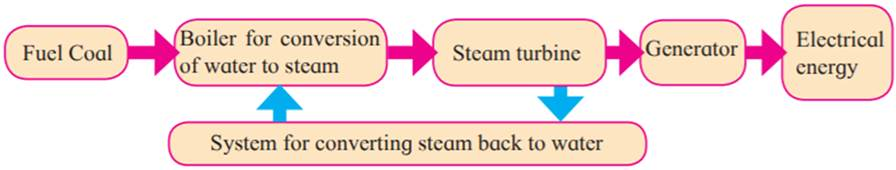Solar thermal electricity generation:

1. The electricity is generated from solar radiations.

2. Reflectors reflect and concentrate solar radiation on absorbers. The solar energy is converted into heat energy, which is used to drive the turbine and generator hence producing electricity.

3.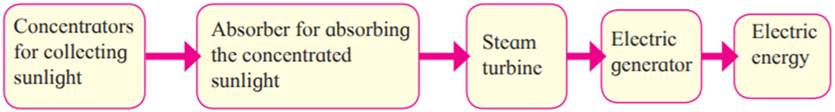Question 8.

What is meant by green energy? Which energy sources can be called as green energy sources and why? Give examples.

i. Electricity made from renewable sources of energy is called green energy.

ii. Green energy is environmental friendly as it does not contribute to depletion of environment.

iii. Green energy can be obtained from sources like wind, tides, sunlight etc. these are called green energy sources.

iv. These are called so, because they are ecofriendly sources of energy which do not cause pollution.

v. Energy sources used are renewable and inexhaustible, which means that they are naturally replenished in nature over a period of time.

Question 9.

Explain the following sentences

Energy obtained from fossil fuels is not green energy.

1. Green energy is environmental friendly energy which does not contribute in degrading the environment. Some examples of green energy sources are wind, sunlight etc. while some examples of fossil fuels are coal, petroleum, natural gas etc.

2. Electricity generation based on fossil fuels like coal, natural gas and nuclear fuels are not environment friendly. Electrical energy generated using these fuels can lead to degradation of the environment.

3. Burning of fossil fuels lead to the emission of toxic gases and soot particles which mix in air causing air pollution and other respiratory diseases.

4. It took millions of years for the formation of these sources. The amount of these fuel available is limited and thus must be used wisely and should not be wasted.

5. Using nuclear fuels, can cause problems regarding the disposal of waste and the possibilities of disasters due to accidents.

According to these facts, the energy generation from fossil fuels is not environment friendly.

Question 10.

Explain the following sentences

Saving energy is the need of the hour.

Conserving energy is the need of the hour because the sources that provide energy for the generation of electricity are being depleted faster than they are being regenerated. The energy resources are being over-consumed. Also, using non-renewable energy sources have a negative impact on the environment. If this problem is overlooked, the future generations will be deprived of electricity, as the non-renewable resources of energy are exhaustible and cannot be replenished naturally. Each and every individual should come forward and contribute to saving energy. By improving on our day to day habits of using electricity, like properly managing electrical appliances, one can reduce the cost of their electricity bills which as an end result can help in conserving energy too.

Question 11.

a. How can we get the required amount of energy by connecting solar panels?[T1]

We can get the required amount of energy by connecting solar photovoltaic cells which convert the solar energy directly into electrical energy. This method is called solar photovoltaic effect.

We get DC supply from the solar panels. Thus, equipment that require DC supply can be directly used whereas for those appliances which demand AC supply, the DC solar power is converted into AC power using an inverter.

These solar panels are semiconductors, usually made out of silicon.

A silicon solar cell of dimension 1 square cm generates current of about 30mA and potential difference of about 0.5V.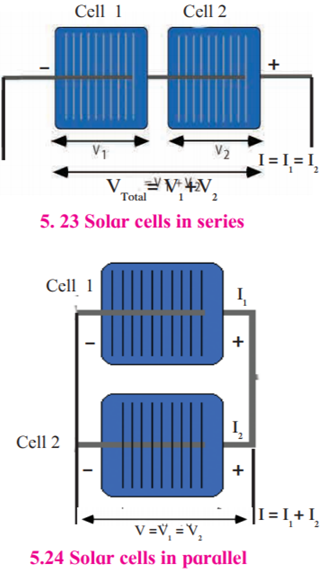Series connection of solar cells: The potential difference obtained from this combination is addition of the potential differences of individual solar cells. However, the current generated from this combination is equal to the current from an individual cell.

Parallel connection of solar cells: If two solar cells are connected in parallel, the current generated from this combination is the summation of the currents from an individual solar cells. However, the potential difference obtained from this combination is the same as the potential difference obtained from individual cell.

Solar panels are connected in series and parallel to generate required DC current and potential difference.

Many solar panels connected in series form solar strings and solar strings connected in parallel form a solar array. Thus, required electrical power is obtained and is used in applications which need marginal power to power station of MW capacity.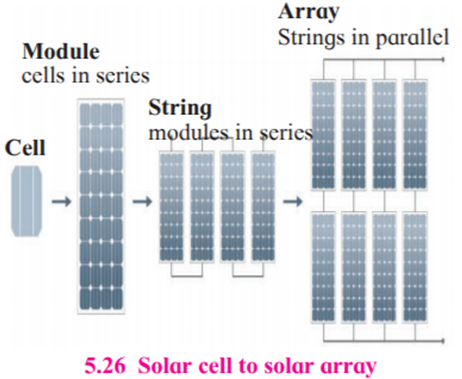Question 12.

What are the advantages and limitations of solar energy?

1. Renewable energy source -solar energy obtained is a renewable source of energy. It is inexhaustible.

2. Produces clean, green power. It does not cause pollution and doesn’t contribute in environment degradation.

1. Production cost of solar cells is very high. Thus, producing and storing electricity via solar energy is indeed an expensive procedure.

2. Low efficiency and Loss of power at night and in bad weather. Solar energy cannot be collected during the night.

Question 13.

Explain with diagram step-by-step energy conversion in

Thermal power plant:

Thermal energy is used here to generate electrical energy; such power plants are called thermal power plants.

In thermal power plants, the chemical energy in the coal is converted into thermal energy. The kinetic energy hence present in the steam is converted to kinetic energy in the turbine. The turbine rotates producing electrical energy.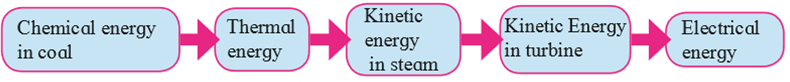Question 14.

Explain with diagram step-by-step energy conversion in

Nuclear Power Plant:

Here nuclear energy is converted into thermal energy, thermal energy is converted into kinetic energy of steam, kinetic energy of steam is converted into kinetic energy of turbine and finally the kinetic energy of the turbine is converted into electrical energy .The step-by-step transformation of energy is shown in the diagram below: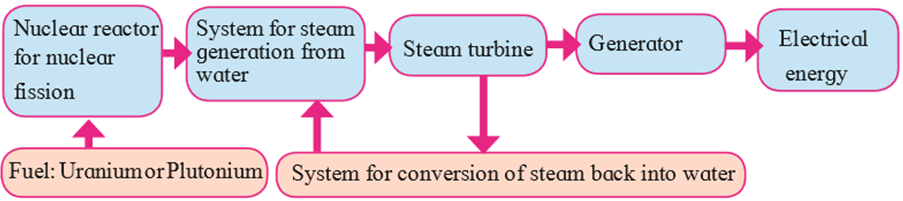Question 15.

Explain with diagram step-by-step energy conversion in

Hydroelectric power plant: